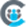# Catch-up on Rules Engine Enhancements

N/A

### July 2020: 6.16 Release

Rules Engine is designed to improve your underlying customer data to produce superior customer outcomes. It can perform powerful multi-step, in-memory data transformations for large datasets in Gainsight within one rule. Transformations include aggregations, multi-dataset merges, formulas and time-series calculations; helping your team to better identify meaningful data signals.

####Improved Rules Engine Formula Functions

Gainsight introduces new Number Formulas to the Formula Fields in Rules Engine. These enable you to perform powerful calculations in your Transformation Tasks while setting up a rule.

The following number formulas are available:

• Abs: The Abs formula returns the absolute value of a number. You can provide your input in the formula field as a positive or negative integer, but the output is always a 7.3890561positive integer. For more information on using the Abs formula, refer to the ABS function article.
• Ceiling: The Ceiling formula maps a number to the least integer greater than or equal to the number. For more information on using the Ceiling formula, refer to the Ceiling function article.
• Exp: The Exp formula returns Euler's number, e (~2.718) raised to a power.
Example: EXP(1) = Approximate value of e = 2.71828183
​​​​EXP(2) = Base of the natural logarithm e raised to the power of 2 = 7.3890561
• Floor: The Floor formula maps a number to the greatest integer less than or equal to the number.  For more information on using the Floor formula, refer to the Floor Function article.
• Log: The Log formula returns the logarithm of a number given a base. For more information on using the Log formula, refer to the Log Function article.
• Sqrt: The Sqrt formula returns the positive square root of a positive number.
• In: The ln formula returns the natural logarithm of a number, base e (Euler's number).
For more information on using the ln formula, refer to the LN function article.Note: For Sqrt, Log, and ln formulas require positive integers as input values. However, the rule runs successfully and no error message is thrown for negative input.

If you enter negative values, these formulas return the following values:

Formula Value
Sqrt zero
In zero
Log null

####Fields in Rules Add/Subtract Date Formula Fields

The Add/Subtract Date Formula field in the Date Formulas of Transformation Tasks now accepts dynamic fields. Previously, this field accepted only static numeric values as input. With this enhancement, the number of days you add to or subtract from a date dynamically changes with the value of the selected field.
Note: You can select the unit of the field as Days only. The Weeks option is not available.For more information on the usage of Date Formulas, refer to the Formula Fields in Bionic Rules article.

N/A

### May 2020: 6.14 Release

Rules Engine is designed to improve your underlying customer data to produce superior customer outcomes. It can perform powerful multi-step, in-memory data transformations for large datasets in Gainsight within one rule. Transformations include aggregations, multi-dataset merges, formulas and time-series calculations; helping your team to better identify meaningful data signals.

With the new Load to PX Action type, admins can now push data to Gainsight PX entities using the Rules Engine in Gainsight CS. PX has two entities, an Account entity and a User entity. With the Load to PX Action, CS administrators can build Rules to load values into custom attributes of the PX Account and User entities. This makes the CS - PX integration a bidirectional data sync combined with the PX connector.

IMPORTANT: To start using the Load to PX Action type from the Actions drop down menu, contact Gainsight Support to get this Action Type enabled for you.

Parent Account ID in PX: The Account entity in PX now has a new custom attribute called Parent Account ID that can track the different values of the parent account. With this attribute, you can group different PX accounts that map to the same Company object in CS.